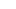Fibonacci is a huge subject and there are many Fibonacci studies with weird-sounding names but we’re going to stick to two: retracement and extension.

## Leonardo Fibonacci

Let us first start by introducing you to the Fib man himself…Leonardo Fibonacci.

Fibonacci was a famous Italian mathematician, also known as a super-duper uber ultra geek.

He had an “Aha!” moment when he discovered a simple series of numbers that created ratios describing the natural proportions of things in the universe.

The ratios arise from the following number series: 0, 1, 1, 2, 3, 5, 8, 13, 21, 34, 55, 89, 144…

This series of numbers are derived by starting with 0 followed by 1 and then adding 0 + 1 to get 1, the third number.

Then, add the second and third numbers (1 + 1) to get 2, the fourth number, and so on.

After the first few numbers in the sequence, if you measure the ratio of any number to the succeeding higher number, you get .618.

## Example

For example, 34 divided by 55 equals .618.

If you measure the ratio between alternate numbers, you get .382.

For example, 34 divided by 89 = 0.382 .

You have now just experienced the Fibonacci Sequence!## Fibonacci Sequence

Fibonacci sequence is formed by taking 2 numbers, any 2 numbers, and adding them together to form a third number.

Then the second and third numbers are added again to form the fourth number.

And you can continue this until it’s not fun anymore.

The ratio of the last number over the second-to-the-last number is approximately equal to 1.618.

This ratio can be found in many natural objects, so this ratio is called the golden ratio.

It appears many times in geometry, art, and architecture.

The golden ratio is actually an irrational number, like pi, and is often denoted by the Greek letter phi (φ).